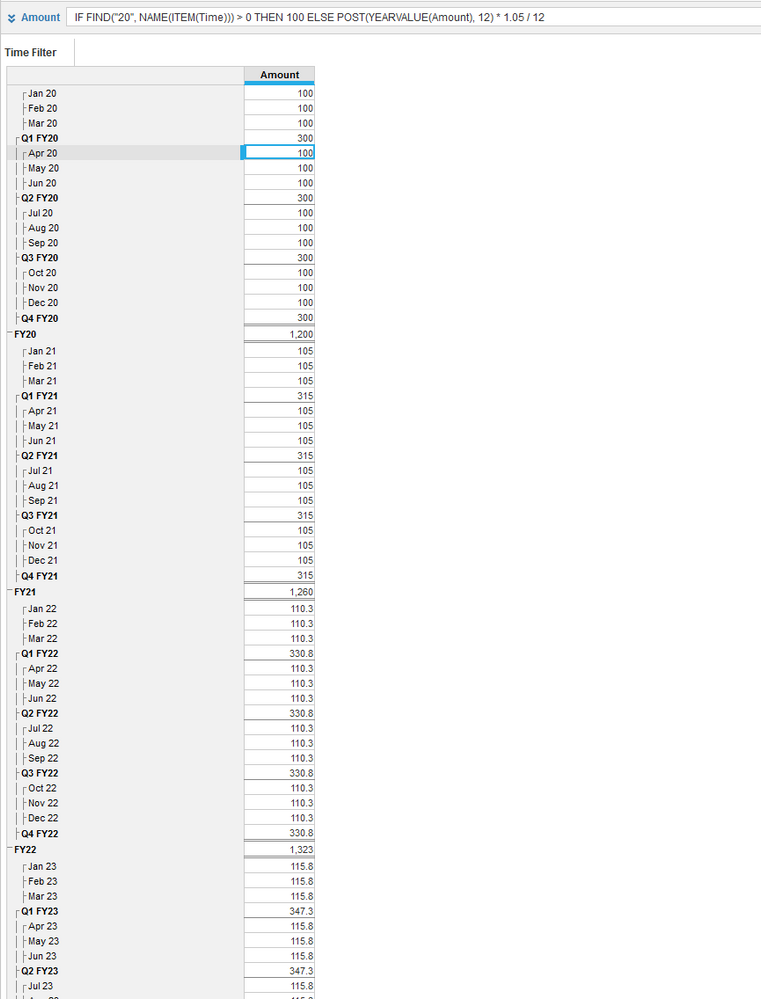# Another Circular Reference QuestionI am building a Cost Forecasting Model which uses different methods for calculating the forecasted amount depending on the cost category and the number of years into the future. One of the latest use cases is to be able to taken the calculated cost from the previous year, increase it by inflation and divide by 12 to give the expected monthly amount for this current year. My problem is getting the total for the previous year, which itself is a product of the calculation. Lookup on year gives a circular reference, so I ended up using "Lag(amount, month no,0,STRICT) + Lag(amount, month no +1 ,0,STRICT)" etc out to +12 where month no is the number of the current month. This effectively fetches the monthly total for each month in the previous year and adds them together. This works but seems overly complex. Is there a better way?

Tagged:

•Hi Jonathan,

Not sure if I fully understood the question

But if you're trying to fetch previous year's data, then apply an inflation factor and divide it by 12, all in the same line item which contains the source data, try this formula.

`POST(YEARVALUE(Amount), 12) * 1.05 / 12`

where amount is the line item containing the source data and also where this formula is being applied

Change 1.05 to the actual inflation factor

Screenshot where I put 100 for each month in 2020 and calculation for months 2021 and onwardsLet me know if this was useful

Regards,

anirudh